Anzeige

# Physics investigatory project class 12th.pdf

3. Jul 2022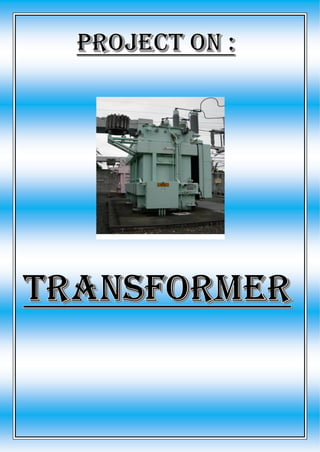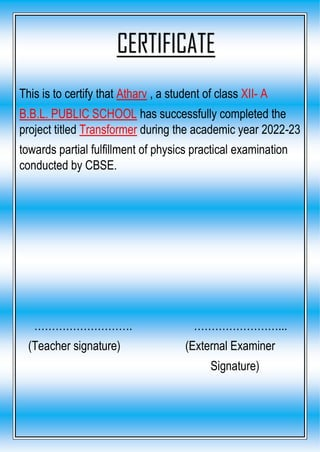Anzeige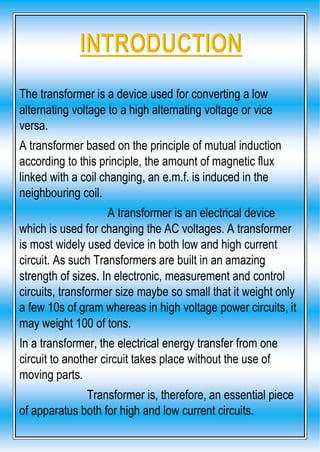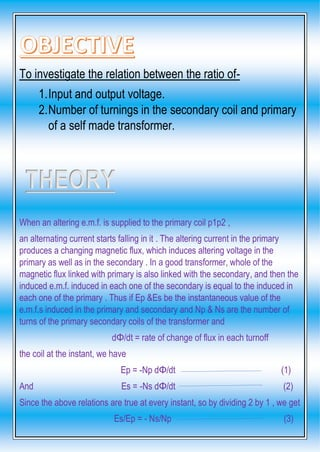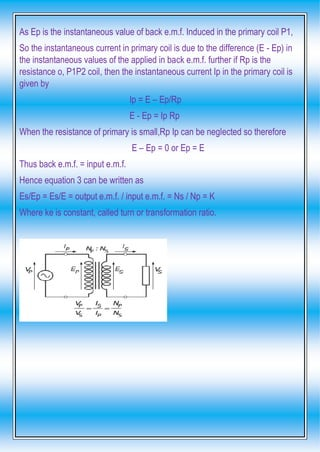Anzeige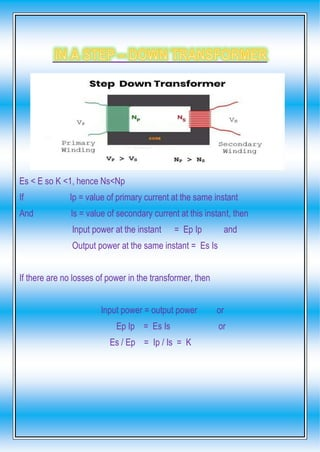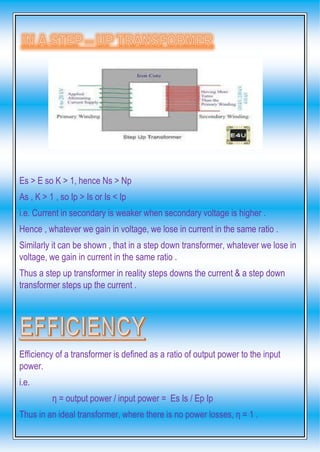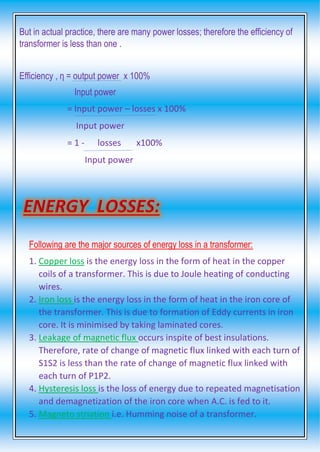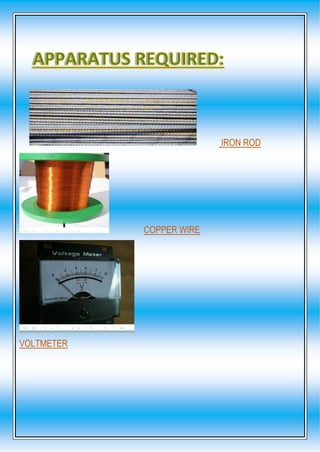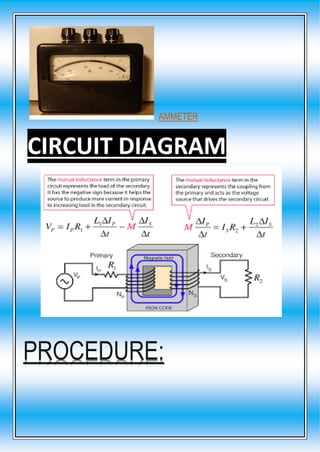Anzeige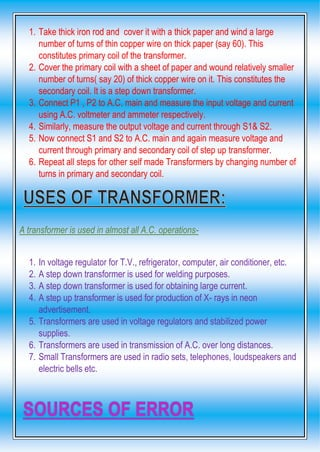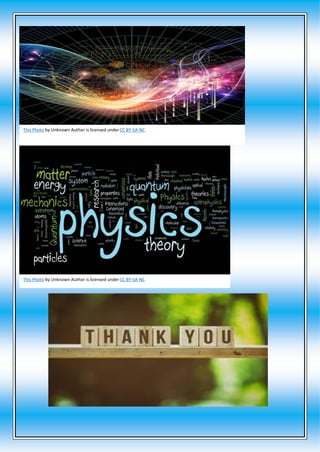Nächste SlideSharePhysics Investigatory Project Class 12
1 von 19
Anzeige

### Physics investigatory project class 12th.pdf

1. MUNDIA AHMED NAGAR, BAREILLY: 243001 Submitted to: submitted by: Saurabh sir atharv Gupta xii- a (2022-23) roll no. - 5
2. Δ CERTIFICATE Δ ACKNOWLEDGEMENT Δ INTRODUCTION Δ THEORY Δ APPRATUS REQUIRED Δ PROCEDURE FOLLOWED Δ OBSERVATION Δ CONCLUSION Δ PRECAUTION Δ BIBLIOGRAPHY
3. This is to certify that Atharv , a student of class XII- A B.B.L. PUBLIC SCHOOL has successfully completed the project titled Transformer during the academic year 2022-23 towards partial fulfillment of physics practical examination conducted by CBSE. ………………………. ……………………... (Teacher signature) (External Examiner Signature) CERTIFICATE
4. I would like to express my special thanks of gratitude to my teacher Mr. Saurabh Gupta as well as our principal Mrs. Alpana Joshi who gave me the golden opportunity to do this wonderful project on the topic transformer which also help me in doing a lot of research and I came to know about so many new things. I’m really thankful to them, Secondly I would also like to thank my parents and friends who helped me a lot in finishing this project within the limited time. I am making this project not only for marks but to also increase my knowledge. THANKS AGAIN TO ALL WHO HELPED ME. ACKNOWLEDGEMENT
5. The transformer is a device used for converting a low alternating voltage to a high alternating voltage or vice versa. A transformer based on the principle of mutual induction according to this principle, the amount of magnetic flux linked with a coil changing, an e.m.f. is induced in the neighbouring coil. A transformer is an electrical device which is used for changing the AC voltages. A transformer is most widely used device in both low and high current circuit. As such Transformers are built in an amazing strength of sizes. In electronic, measurement and control circuits, transformer size maybe so small that it weight only a few 10s of gram whereas in high voltage power circuits, it may weight 100 of tons. In a transformer, the electrical energy transfer from one circuit to another circuit takes place without the use of moving parts. Transformer is, therefore, an essential piece of apparatus both for high and low current circuits.
6. To investigate the relation between the ratio of- 1.Input and output voltage. 2.Number of turnings in the secondary coil and primary of a self made transformer. When an altering e.m.f. is supplied to the primary coil p1p2 , an alternating current starts falling in it . The altering current in the primary produces a changing magnetic flux, which induces altering voltage in the primary as well as in the secondary . In a good transformer, whole of the magnetic flux linked with primary is also linked with the secondary, and then the induced e.m.f. induced in each one of the secondary is equal to the induced in each one of the primary . Thus if Ep &Es be the instantaneous value of the e.m.f.s induced in the primary and secondary and Np & Ns are the number of turns of the primary secondary coils of the transformer and dΦ/dt = rate of change of flux in each turnoff the coil at the instant, we have Ep = -Np dΦ/dt (1) And Es = -Ns dΦ/dt (2) Since the above relations are true at every instant, so by dividing 2 by 1 , we get Es/Ep = - Ns/Np (3) THEORY
7. As Ep is the instantaneous value of back e.m.f. Induced in the primary coil P1, So the instantaneous current in primary coil is due to the difference (E - Ep) in the instantaneous values of the applied in back e.m.f. further if Rp is the resistance o, P1P2 coil, then the instantaneous current Ip in the primary coil is given by Ip = E – Ep/Rp E - Ep = Ip Rp When the resistance of primary is small,Rp Ip can be neglected so therefore E – Ep = 0 or Ep = E Thus back e.m.f. = input e.m.f. Hence equation 3 can be written as Es/Ep = Es/E = output e.m.f. / input e.m.f. = Ns / Np = K Where ke is constant, called turn or transformation ratio.
8. Es < E so K <1, hence Ns<Np If Ip = value of primary current at the same instant And Is = value of secondary current at this instant, then Input power at the instant = Ep Ip and Output power at the same instant = Es Is If there are no losses of power in the transformer, then Input power = output power or Ep Ip = Es Is or Es / Ep = Ip / Is = K
9. Es > E so K > 1, hence Ns > Np As , K > 1 , so Ip > Is or Is < Ip i.e. Current in secondary is weaker when secondary voltage is higher . Hence , whatever we gain in voltage, we lose in current in the same ratio . Similarly it can be shown , that in a step down transformer, whatever we lose in voltage, we gain in current in the same ratio . Thus a step up transformer in reality steps downs the current & a step down transformer steps up the current . Efficiency of a transformer is defined as a ratio of output power to the input power. i.e. η = output power / input power = Es Is / Ep Ip Thus in an ideal transformer, where there is no power losses, η = 1 .
10. But in actual practice, there are many power losses; therefore the efficiency of transformer is less than one . Efficiency , η = output power x 100% Input power = Input power – losses x 100% Input power = 1 - losses x100% Input power Following are the major sources of energy loss in a transformer: 1. Copper loss is the energy loss in the form of heat in the copper coils of a transformer. This is due to Joule heating of conducting wires. 2. Iron loss is the energy loss in the form of heat in the iron core of the transformer. This is due to formation of Eddy currents in iron core. It is minimised by taking laminated cores. 3. Leakage of magnetic flux occurs inspite of best insulations. Therefore, rate of change of magnetic flux linked with each turn of S1S2 is less than the rate of change of magnetic flux linked with each turn of P1P2. 4. Hysteresis loss is the loss of energy due to repeated magnetisation and demagnetization of the iron core when A.C. is fed to it. 5. Magneto striation i.e. Humming noise of a transformer.